Next: A model for convolution Up: GEOMETRY-BASED DECON Previous: Signature

## Regressing simultaneously before and after NMO

Before launching into a complicated theory for suppressing both reverberation and signature, let us make some guesses. Let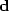denote an original data panel like the left sides of Figure 7 and 8, and let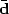be moved out like the right sides of those figures. If we had only signature to contend with, we might formulate the problem as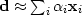, where theare delayed versions of the data, containing,and where the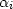are the scaling coefficients to be found. If we had only reverberation to contend with, we might formulate the problem as, where the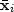are delayed versions of the moved-out data, and the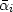are more unknowns.

To suppress both signature and reverberation simultaneously, we need to express both statements of wishes'' in the same domain, either moved out or not. Lettingbe the moveout operator, and choosing the moved-out domain, we write the statement of wishes as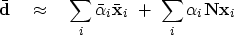(1)

Why not estimate the filters sequentially instead of simultaneously? What fails if we first process raw data by blind deconvolution for the source signature, then do NMO, and finally do blind deconvolution again for reverberation?

At vertical incidence, both filters are convolutional, and they are indistinguishable. At vertical incidence, doing a stage of deconvolution for each process leads to nonsensical answers. Whichever stage is applied first will absorb all the color in the data, leaving nothing for the second stage. The color will not be properly distributed between the stages. In principle, at nonzero offset the information is present to distinguish between the stages, but the first stage will always tend to absorb the color attributable to both. A simpler expression of the same concept arises when we are regressing two theoretical signals against some data. If the regressors are orthogonal, such as a mean value and a sinusoid, then we tend to get the same result regardless of the order in which we subtract them from the signal. If the regressors resemble one another, as a mean can resemble a trend, then they must be estimated simultaneously.Next: A model for convolution Up: GEOMETRY-BASED DECON Previous: Signature
Stanford Exploration Project
10/21/1998